## Calculation of the conjugate of piecewise functions

#### Conjugate of piecewise function

In this post, we’ll show how to calculate the conjugate of a piecewise function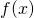,
such as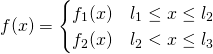The conjugate for the above is: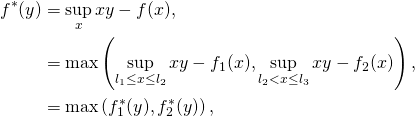whereis the conjugate of the function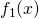constrained to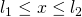. So, computing the conjugate of a piecewise function is simple as long as the conjugates of the sub-functions can be computed easily.

#### Conjugate of constrained function

In this section, we’ll show how to calculate the convex conjugate of a function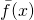, which is the functionwhich is constrained to a domain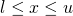.
The conjugate is defined as: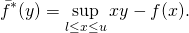For the unconstrained case, the solution would be given by: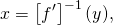where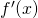is the derivative of. Sinceis convex,is a monotonically increasing function. Therefore, we haveThe conjugate of the constrained function is therefore: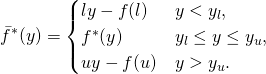The convex conjugate for the unconstrained function can be automatically calculated in Python — as described in the previous post.

Code that do the above is given below:

def calc_const_conj(fun_str, lstr='l', ustr='u', varnames='x l u', fname='plot.png'):
# set the symbols
vars = sp.symbols(varnames)
x = vars if isinstance(vars, tuple) else vars
y = sp.symbols('y', real=True)

# set the function and objective
fun = parse_expr(fun_str)
obj = x*y - fun
fun_diff = sp.diff(fun, x)
obj_diff = sp.diff(obj, x)

l = parse_expr(lstr)
u = parse_expr(ustr)

# calculate yl and yu
yl = sp.simplify(fun.subs(x, l))
yu = sp.simplify(fun.subs(x, u))

dyl = sp.simplify(fun_diff.subs(x, l))
dyu = sp.simplify(fun_diff.subs(x, u))

# calculate derivative of obj and solve for zero
sol = solve(obj_diff, x)

# substitute solution into objective
solfun = sp.collect(sp.simplify(obj.subs(x, sol)), x)

# print the function and conjugate:
print('{0:45} y < {1}'.format(str(sp.simplify(l*y - yl)), dyl))
print('{0:45} {1} <= y <= {2}'.format(str(solfun), dyl, dyu))
print('{0:45} y > {1}'.format(str(sp.simplify(u*y - yu)), dyu))

# Example: calc_const_conj('1/2*x**2', lstr='-1', ustr='1', varnames='x')


#### Table of conjugates

 Function Constraint Conjugate Linear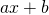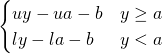Simple Quadratic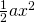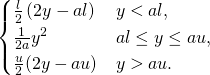Quadratic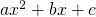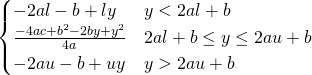PE-like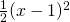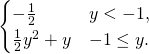Hinge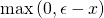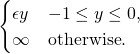(scaled)Hinge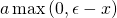## Automatically calculating the convex conjugate (Fenchel dual) using Python/Sympy

The convex conjugate (or Fenchel dual) for a single variable functionis defined as: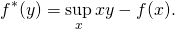We assume thatis convex, differentiable and simple (closed-form solution exist for minimum). The conjugate can then symbolically be calculated using the following Python code:

def calc_conjugate(str, varnames='x'):

# set the symbols
vars = sp.symbols(varnames)
x = vars if isinstance(vars, tuple) else vars
y = sp.symbols('y', real=True)

# set the function and objective
fun = parse_expr(str)
obj = x*y - fun

# calculate derivative of obj and solve for zero
sol = solve(sp.diff(obj, x), x)

# substitute solution into objective
solfun = sp.simplify(obj.subs(x, sol))

# if extra values were passed add to lambda function
varnames = [y] + list(vars[1:]) if isinstance(vars, tuple) else y

return (sp.sstr(solfun), lambdify(vars, fun, 'numpy'), lambdify(varnames, solfun, 'numpy'))


The full source code is here. The above code was used to calculate and plot the conjugates of several functions, which is given below.

 Function Conjugate Quadratic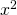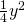Exponential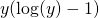Log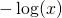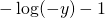Log-Exponential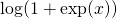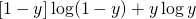x log(x)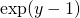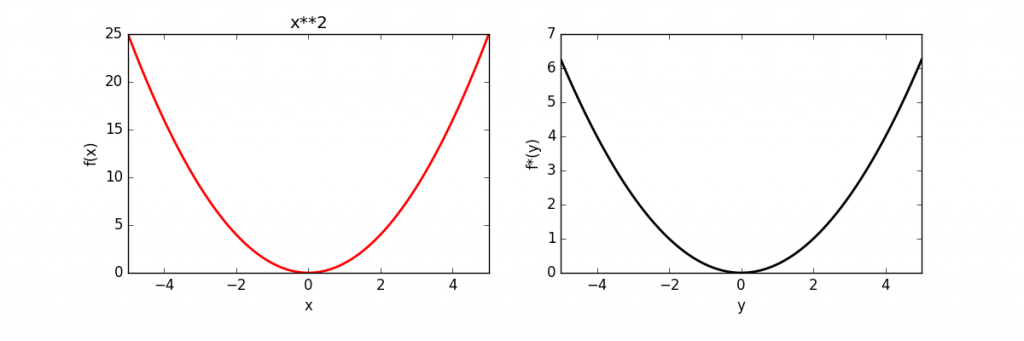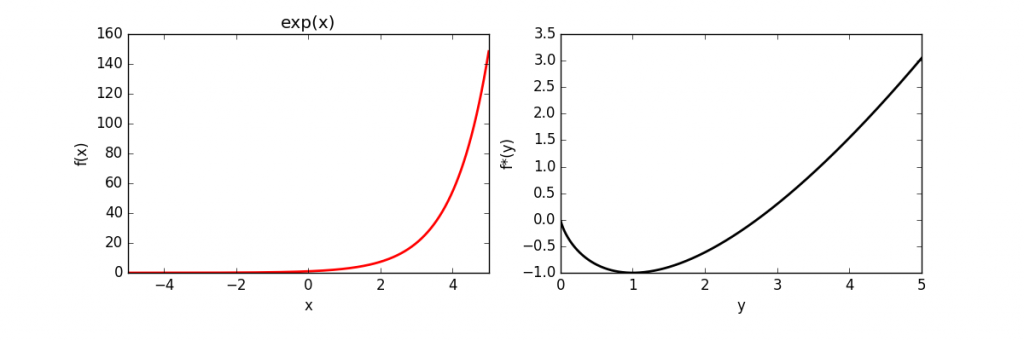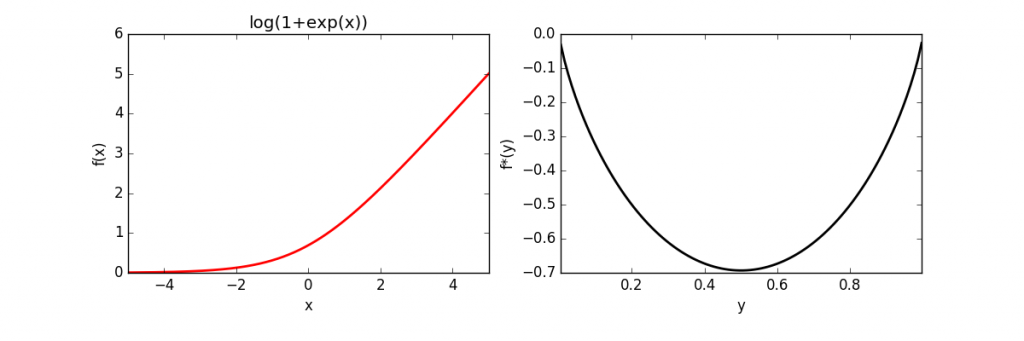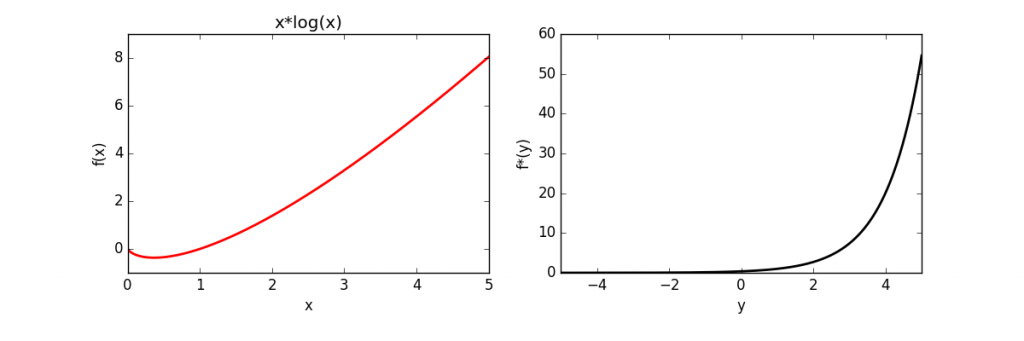## Fast projection onto a Simplex (Python)

(work in progress)

Many convex optimization problems can be solved, fairly simply, if the projection onto the constraints can be quickly and simply calculated. By projection onto the constraints, we mean the solution to the following optimization problem:

(1)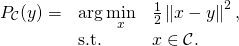As an example, the subgradient descent method can incorporate the projection operator to deal with constraints. Here we focus on projection on a simplex: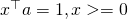. The objective function that we wish to solve is:

(2)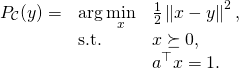As a test case, we can implement the projection in cvxpy:

import cvxpy as cvx
import numpy as np

def proj_simplex_cvxpy(a, y):
'''
Returns the point in the simplex a^Tx = 1, x&amp;amp;amp;amp;gt;=0 that is
closest to y (according to Euclidian distance)
'''
d = len(a)

# setup the objective and constraints and solve the problem
x = cvx.Variable(d)
obj = cvx.Minimize(cvx.sum_squares(x - y))
constr = [x &gt;= 0, a*x == 1]
prob = cvx.Problem(obj, constr)
prob.solve()

return np.array(x.value).squeeze()


We can write the dual as:Since the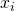decouples (for fixed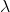) it can be solved as: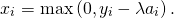The dual objective (which is to be maximized) is therefore:An example of the dual function is given below (source code is here):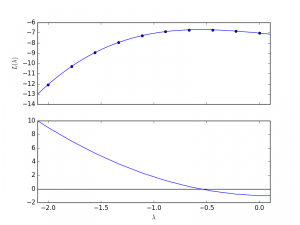Asis decreased from a large value, the values ofwill “switch on” from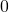. To determine where the variables will switch on we sort the values according to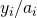.
Consider that we have an index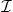that sorts according to. In other words: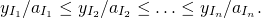We can refer to these as our indexedvalues,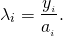Suppose that we have avalue. We then want to find an index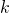, so that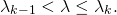We can then write the objective function as: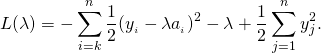The derivative of the above is therefore: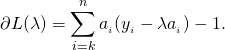By searching on the sorted list, we get an index, such that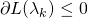, but.
The optimal lambda value can then be calculated as: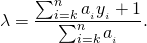The final algorithm is:

def proj(a, y):

l = y/a
idx = np.argsort(l)
d = len(l)

evalpL = lambda k: np.sum(a[idx[k:]]*(y[idx[k:]] - l[idx[k]]*a[idx[k:]]) ) -1

def bisectsearch():
idxL, idxH = 0, d-1
L = evalpL(idxL)
H = evalpL(idxH)

if L<0:
return idxL

while (idxH-idxL)>1:
iMid = int((idxL+idxH)/2)
M = evalpL(iMid)

if M>0:
idxL, L = iMid, M
else:
idxH, H = iMid, M

return idxH

k = bisectsearch()
lam = (np.sum(a[idx[k:]]*y[idx[k:]])-1)/np.sum(a[idx[k:]])

x = np.maximum(0, y-lam*a)

return x


## Other resources:

• MATLAB implementation of projection onto unit simplex (description here)
• Michelot, C. (1986). A finite algorithm for finding the projection of a point onto the canonical simplex of∝ n. Journal of Optimization Theory and Applications, 50(1), 195-200.

### Todo:

1. Implement the code in C/C++
2. Give a better test case
3. Simplify the explanation and implementation
4. Give a practical example (e.g. estimation problem with subgradient descent)

## Topics (to be done in coming weeks)

1. Projection on a simplex (with Python implementation)
2. Speed comparison between quadratic programming solvers of Gurobi and cvxpy using SVM as baseline
3. Python implementation of robust SVM (with ramp loss) using the ConCave-Convex Procedure

Latex test: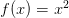$f(x) = x^2$